# Runtime Workaround #2: How to Combine Marker Symbol Layers

347
2
05-02-2019 02:40 PM
Regular Contributor
0 2 347

In my previous article, I presented a workaround for preserving group layers in a mobile map opened using ArcGIS Runtime SDK for .NET 100.5.  Today's topic involves something a bit nastier.  It can be pretty frustrating when a bug that is fixed in an earlier software version reappears in a later one.  The lesson here is: Never discard your workaround code!

The bug in question involves certain multi-layer marker symbols that are not rendered properly when rotated.  For example, see this symbol as shown in the original ArcGIS Pro project:Here's how it looks when exported to a mobile map package and opened using ArcGIS Runtime (see the attached Visual Studio example project):Yikes!  This problem was identified at 100.1 and fixed in 100.2, but at 100.5 once more it rears its ugly head.  One workaround is to set ArcGISFeatureTable.UseAdvancedSymbology to false.  This causes marker symbols to be rendered as picture markers.  That's fine until you run into two limitations.  The first is when you set a reference scale and zoom in: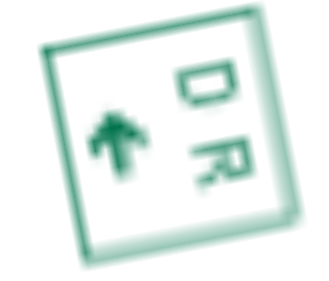But even more challenging, what if you want to change symbol colors on the fly?  In theory, you can do that with a bitmap, but it's beyond my skill to deal with the dithering: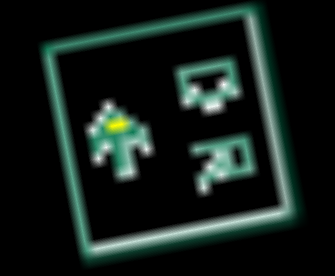There's another approach, but until Esri implements more fine-grained class properties and methods, manipulating symbols involves a lot of JSON hacking.  Before I go any further, let's crack open a mobile map package and see where drawing information is stored.  If you examine the mobile geodatabase using a tool such as SQLiteSpy,

you will see a table called GDB_ServiceItems: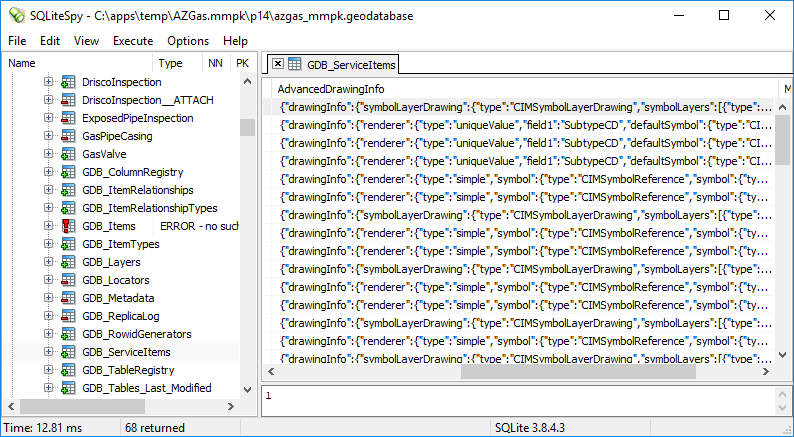That's the raw JSON for the data retrieved by ArcGISFeatureTable.LayerInfo.DrawingInfo.  Fortunately, there's no need to hack into the table, because you can get the renderer for a feature layer, retrieve the symbol(s), and convert them to JSON.  Then you make whatever edits you want, and create a new symbol.

``		public static Symbol UpdateSymbolJSON(MultilayerPointSymbol symOld, Color colorOld, Color colorNew)		{			string sOldJSON = symOld.ToJson();			Dictionary<string, object> dict = (Dictionary<string, object>)_js.DeserializeObject(sOldJSON);			SymbolHelper.ProcessObjectColorJSON(dict, colorOld, colorNew);			string sNewJSON = _js.Serialize(dict);			Symbol symNew = Symbol.FromJson(sNewJSON);			return symNew;		}‍‍‍‍‍‍‍‍‍‍‍‍‍‍‍‍‍‍‍``

So what's the workaround?  The nature of the bug seems to be an inability to process offsetX and offsetY correctly.  In fact, they seem to be reversed.  So let's see what happens when the offsets are reversed in the JSON: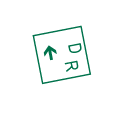Nope.  Not quite there.  What I finally ended up doing is to combine the offset layers into a single layer with no offsets.  Fortunately again, characters are already converted to polygons in the JSON, or I would be doing a lot more work.  First, I collect the offset layers and find the smallest interval (points per coordinate unit):

``			bool[] Offset = new bool[layers.Length];			List<OffsetLayer> OffsetLayers = new List<OffsetLayer>();			double dInterval = double.MaxValue;			for (int i = 0; i < layers.Length; i++)			{				Dictionary<string, object> lyr = layers[i] as Dictionary<string, object>;				// Check for X and/or Y offset				bool bOffset = false;				double dOffsetX = 0;				double dOffsetY = 0;				if (lyr.ContainsKey("offsetX"))				{					dOffsetX = Convert.ToDouble(lyr["offsetX"]);					lyr["offsetX"] = 0;					bOffset = true;				}				if (lyr.ContainsKey("offsetY"))				{					dOffsetY = Convert.ToDouble(lyr["offsetY"]);					lyr["offsetY"] = 0;					bOffset = true;				}				Offset[i] = bOffset;				if (!bOffset)					continue;				// Get offset layer data				Dictionary<string, object> frame = lyr["frame"] as Dictionary<string, object>;				object[] markerGraphics = lyr["markerGraphics"] as object[];				Dictionary<string, object> markerGraphic = markerGraphics as Dictionary<string, object>;				Dictionary<string, object> geometry = markerGraphic["geometry"] as Dictionary<string, object>;				object[] rings = geometry["rings"] as object[];				int ymin = Convert.ToInt32(frame["ymin"]);				int ymax = Convert.ToInt32(frame["ymax"]);				double size = Convert.ToDouble(lyr["size"]);				double dInt = size / (ymax - ymin);				if (dInt < dInterval)					dInterval = dInt;				OffsetLayer layer = new OffsetLayer()				{					offsetX = dOffsetX,					offsetY = dOffsetY,					xmin = Convert.ToInt32(frame["xmin"]),					ymin = ymin,					xmax = Convert.ToInt32(frame["xmax"]),					ymax = ymax,					size = size,					rings = rings				};				OffsetLayers.Add(layer);			} // for‍‍‍‍‍‍‍‍‍‍‍‍‍‍‍‍‍‍‍‍‍‍‍‍‍‍‍‍‍‍‍‍‍‍‍‍‍‍‍‍‍‍‍‍‍‍‍‍‍‍‍‍‍‍‍‍‍‍‍‍‍‍‍‍‍‍‍‍‍‍‍‍‍‍‍‍‍‍‍‍‍‍‍‍‍‍‍‍‍‍‍‍‍‍‍‍‍‍‍‍‍‍‍‍‍‍‍‍‍‍‍‍‍‍‍``

Then I set up the combined frame and recalculate the ring coordinates:

``			int iMinX = 0;			int iMinY = 0;			int iMaxX = 0;			int iMaxY = 0;			List<object[]> OffsetRings = new List<object[]>();			foreach (OffsetLayer lyr in OffsetLayers)			{				double dX, dY;				int iX, iY;				// Set up transformation				double dInt = lyr.size / (lyr.ymax - lyr.ymin);				double dOffsetX = lyr.offsetX / dInt;				double dOffsetY = lyr.offsetY / dInt;				double dScale = dInt / dInterval;				dX = (lyr.xmin + dOffsetX) * dScale;				iX = (int)dX;				if (iX < iMinX)					iMinX = iX;				dX = (lyr.xmax + dOffsetX) * dScale;				iX = (int)dX;				if (iX > iMaxX)					iMaxX = iX;				dY = (lyr.ymin + dOffsetY) * dScale;				iY = (int)dY;				if (iY < iMinY)					iMinY = iY;				dY = (lyr.ymax + dOffsetY) * dScale;				iY = (int)dY;				if (iY > iMaxY)					iMaxY = iY;				// Recalculate rings				foreach (object obj in lyr.rings)				{					object[] ring = obj as object[];					foreach (object o in ring)					{						object[] pt = o as object[];						pt = (int)((Convert.ToInt32(pt) + dOffsetX) * dScale);						pt = (int)((Convert.ToInt32(pt) + dOffsetY) * dScale);					}					OffsetRings.Add(ring);				}			} // foreach			double dSize = (iMaxY - iMinY) * dInterval;‍‍‍‍‍‍‍‍‍‍‍‍‍‍‍‍‍‍‍‍‍‍‍‍‍‍‍‍‍‍‍‍‍‍‍‍‍‍‍‍‍‍‍‍‍‍‍‍‍‍‍‍‍‍‍‍‍‍‍‍‍‍‍‍‍‍‍‍‍‍‍‍‍‍‍‍‍‍‍‍‍‍‍‍‍‍‍‍‍‍‍‍‍‍‍‍‍‍‍‍‍‍‍‍‍‍‍‍‍‍‍‍‍‍‍‍‍‍‍‍‍‍‍‍‍‍‍‍‍‍‍‍‍‍‍‍‍‍‍‍‍‍‍‍‍‍‍‍‍‍‍‍``

Finally, I assemble a new symbol layer list:

``			List<object> NewLayers = new List<object>();			bool bFirst = true;			for (int i = 0; i < layers.Length; i++)			{				if (!Offset[i])				{					NewLayers.Add(layers[i]);					continue;				}				else if (!bFirst)					continue;				// Update first offset layer				Dictionary<string, object> lyr = layers[i] as Dictionary<string, object>;				Dictionary<string, object> frame = lyr["frame"] as Dictionary<string, object>;				frame["xmin"] = iMinX;				frame["ymin"] = iMinY;				frame["xmax"] = iMaxX;				frame["ymax"] = iMaxY;				lyr["size"] = dSize;				if (lyr.ContainsKey("offsetX"))					lyr["offsetX"] = 0;				if (lyr.ContainsKey("offsetY"))					lyr["offsetY"] = 0;				NewLayers.Add(lyr);				object[] markerGraphics = lyr["markerGraphics"] as object[];				Dictionary<string, object> markerGraphic = markerGraphics as Dictionary<string, object>;				Dictionary<string, object> geometry = markerGraphic["geometry"] as Dictionary<string, object>;				geometry["rings"] = OffsetRings.ToArray();				bFirst = false;			} // for			return NewLayers.ToArray();‍‍‍‍‍‍‍‍‍‍‍‍‍‍‍‍‍‍‍‍‍‍‍‍‍‍‍‍‍‍‍‍‍‍‍‍‍‍‍‍‍‍‍‍‍‍‍‍‍‍‍‍‍‍‍‍‍‍‍‍‍‍‍‍‍‍‍‍‍‍‍``

And here are the results: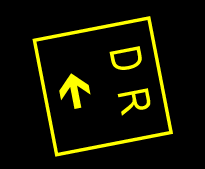Much better.  I can't guarantee that this code will work for every situation, but it seems to work fine for my own complex symbols.  And remember:  even if this bug is fixed at 100.6, hang onto this code, in case you need it again in the future!